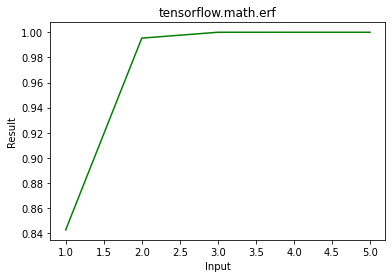# Python – tensorflow.math.erf()

TensorFlow is open-source Python library designed by Google to develop Machine Learning models and deep learning  neural networks.

erf() is used to compute element wise Gauss error function.

Syntax: tensorflow.math.erf(   x, name)

Parameters:

• x: It’s the input tensor. Allowed dtypes are bfloat16, half, float32, float64.
• name(optional): It defines the name for the operation.

Returns: It returns a tensor of same dtype as x.

Example 1:

## Python3

 `# importing the library ` `import` `tensorflow as tf ` ` `  `# Initializing the input tensor ` `a ``=` `tf.constant([``1``, ``2``, ``3``, ``4``, ``5``], dtype ``=` `tf.float64) ` ` `  `# Printing the input tensor ` `print``(``'Input: '``, a) ` ` `  `# Calculating Gauss error ` `res ``=` `tf.math.erf(x ``=` `a) ` ` `  `# Printing the result ` `print``(``'Result: '``, res) `

Output:

```Input:  tf.Tensor([1. 2. 3. 4. 5.], shape=(5, ), dtype=float64)
Result:  tf.Tensor([0.84270079 0.99532227 0.99997791 0.99999998 1.        ], shape=(5, ), dtype=float64)

```

Example 2: Visualization

## Python3

 `# importing the library ` `import` `tensorflow as tf ` `import` `matplotlib.pyplot as plt ` ` `  `# Initializing the input tensor ` `a ``=` `tf.constant([``1``, ``2``, ``3``, ``4``, ``5``], dtype ``=` `tf.float64) ` ` `  `# Calculating Gauss error ` `res ``=` `tf.math.erf(x ``=` `a) ` ` `  `# Plotting the graph ` `plt.plot(a, res, color ``=``'green'``) ` `plt.title(``'tensorflow.math.erf'``) ` `plt.xlabel(``'Input'``) ` `plt.ylabel(``'Result'``) ` `plt.show() `

Output:My Personal Notes arrow_drop_upCheck out this Author's contributed articles.

If you like GeeksforGeeks and would like to contribute, you can also write an article using contribute.geeksforgeeks.org or mail your article to contribute@geeksforgeeks.org. See your article appearing on the GeeksforGeeks main page and help other Geeks.

Please Improve this article if you find anything incorrect by clicking on the "Improve Article" button below.

Article Tags :

Be the First to upvote.

Please write to us at contribute@geeksforgeeks.org to report any issue with the above content.